# Zeckendorf representation

(diff) ← Older revision | Latest revision (diff) | Newer revision → (diff)

Every positive integercan be expressed uniquely as a sum of distinct non-consecutive Fibonacci numbers. This result is called Zeckendorf's theorem and the sequence of Fibonacci numbers which add up tois called the Zeckendorf representation of. The theorem and the representation are named after the Belgian medical doctor and amateur mathematician E. Zeckendorf (1901–1983). See [a3] for a fine brief biography of Zeckendorf.

The Fibonacci sequence is defined by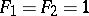andfor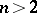. The precise sequence used in the Zeckendorf theorem and representation is the Fibonacci sequence withdeleted, the first few members being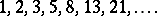Examples of Zeckendorf representations are:To construct the Zeckendorf representation of, one chooses the largest Fibonacci number not greater than, say, and subtracts it from. Unlessis thereby reduced to zero, one then finds the largest Fibonacci number not greater than, say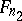, and subtracts it, etc. Ifis reduced to zero aftersteps, one obtains a Zeckendorf representation of the formwhere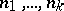is a decreasing sequence of positive integers. This representation cannot include two consecutive Fibonacci numbers, say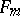and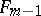, for this would imply that their sum,, or some larger Fibonacci number should have been chosen in place of. The smallest integer whose Zeckendorf representation is the sum ofFibonacci numbers is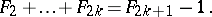It can be shown by construction that no other sequence can be substituted for the Fibonacci sequence in the statement of Zeckendorf's theorem (see [a1]). Fibonacci numbers have been known to mathematics for some 800 years and it seems rather surprising that this property of them did not receive attention until relatively recently. Indeed, nothing appeared in print concerning the Zeckendorf representation until the middle of the 20th century, with the publication of a paper of C.G. Lekkerkerker [a4]. Zeckendorf did not publish his own account until 1972 (see [a6]) although (see [a3]) he had a proof of his theorem by 1939. Every positive integer can also be uniquely represented as a sum of different powers of two, and it is natural to compare Zeckendorf representations with binary representations. This is pursued in [a2], where there is a discussion of algorithms which, given two positive integersandin Zeckendorf form, produce the Zeckendorf forms forand. Even the addition algorithm is rather more complicated than the corresponding algorithm for binary arithmetic. (If this were not so, "Zeckendorf arithmetic" might be used nowadays instead of binary arithmetic.) The Zeckendorf multiplication algorithm given in [a2] is based on the fact that, for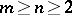, the Zeckendorf representation of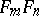isforeven. Whenis odd, one has to add one further term to the above sum: the term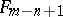whenand the term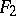when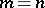. In the upper limit of the summation,denotes the largest integer not greater than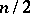.

An amusing trivial "application" of the Zeckendorf representation is a method of converting miles into kilometres and vice versa without having to perform a multiplication. It relies on the coincidence that the number of kilometres in a mile (approximately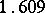) is close to the golden-section number (cf. also Golden ratio),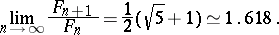Thus, to convert miles into kilometres one writes down the (integer) number of miles in Zeckendorf form and replaces each of the Fibonacci numbers by its successor. This will give the Zeckendorf form of the corresponding approximate number of kilometres. For example,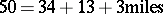is approximately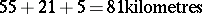andkilometres is approximatelyHow to Cite This Entry:
Zeckendorf representation. Encyclopedia of Mathematics. URL: http://encyclopediaofmath.org/index.php?title=Zeckendorf_representation&oldid=18410
This article was adapted from an original article by G.M. Phillips (originator), which appeared in Encyclopedia of Mathematics - ISBN 1402006098. See original article